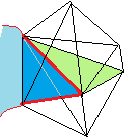CGAL 4.7 - Advancing Front Surface Reconstruction
Advancing Front Surface Reconstruction ReferenceTran Kai Frank Da, David Cohen-Steiner
This package provides a greedy algorithm for surface reconstruction from an unorganized point set. Starting from a seed facet, a piecewise linear surface is grown by adding Delaunay triangles one by one. The most plausible triangles are added first, in a way that avoids the appearance of topological singularities.

Introduced in: CGAL 4.7
Depends on: 3D Triangulations
BibTeX: cgal:dc-afsr-15b
License: GPL

## Classes

• CGAL::Advancing_front_surface_reconstruction
• CGAL::Advancing_front_surface_reconstruction_vertex_base_3
• CGAL::Advancing_front_surface_reconstruction_cell_base_3

## Functions

• CGAL::advancing_front_surface_reconstruction()

## Classes

class  CGAL::Advancing_front_surface_reconstruction< Dt, F >
The class Advancing_front_surface_reconstruction enables advanced users to provide the unstructured point cloud in a 3D Delaunay triangulation. More...

class  CGAL::Advancing_front_surface_reconstruction_cell_base_3< Traits, Cb >
The class Advancing_front_surface_reconstruction_cell_base_3 is the default cell type for the class Advancing_front_surface_reconstruction. More...

class  CGAL::Advancing_front_surface_reconstruction_vertex_base_3< Traits, Vb >
The class Advancing_front_surface_reconstruction_vertex_base_3 is the default vertex type for the class Advancing_front_surface_reconstruction. More...

## Functions

template<typename PointInputIterator , typename IndicesOutputIterator >
IndicesOutputIterator CGAL::advancing_front_surface_reconstruction (PointInputIterator b, PointInputIterator e, IndicesOutputIterator out, double radius_ratio_bound=5, double beta=0.52)
For a sequence of points computes a sequence of index triples describing the faces of the reconstructed surface. More...

template<typename PointInputIterator , typename IndicesOutputIterator , typename Filter >
IndicesOutputIterator CGAL::advancing_front_surface_reconstruction (PointInputIterator b, PointInputIterator e, IndicesOutputIterator out, Filter filter, double radius_ratio_bound=5, double beta=0.52)
For a sequence of points computes a sequence of index triples describing the faces of the reconstructed surface. More...

## Function Documentation

template<typename PointInputIterator , typename IndicesOutputIterator >
 IndicesOutputIterator CGAL::advancing_front_surface_reconstruction ( PointInputIterator b, PointInputIterator e, IndicesOutputIterator out, double radius_ratio_bound = 5, double beta = 0.52 )

For a sequence of points computes a sequence of index triples describing the faces of the reconstructed surface.

Template Parameters
 PointInputIterator must be an input iterator with 3D points as value type. This point type must be convertible to Exact_predicates_inexact_constructions_kernel::Point_3 with the Cartesian_converter. IndicesOutputIterator must be an output iterator to which CGAL::cpp11::tuple can be assigned.
Parameters
 b iterator on the first point of the sequence e past the end iterator of the point sequence out output iterator radius_ratio_bound candidates incident to surface triangles which are not in the beta-wedge are discarded, if the ratio of their radius and the radius of the surface triangle is larger than radius_ratio_bound. Described in Section Dealing with Multiple Components, Boundaries and Sharp Edges beta half the angle of the wedge in which only the radius of triangles counts for the plausibility of candidates. Described in Section Plausibility of a Candidate Triangle

#include <CGAL/Advancing_front_surface_reconstruction.h>

Examples:
Advancing_front_surface_reconstruction/reconstruction_fct.cpp, and Advancing_front_surface_reconstruction/reconstruction_surface_mesh.cpp.
template<typename PointInputIterator , typename IndicesOutputIterator , typename Filter >
 IndicesOutputIterator CGAL::advancing_front_surface_reconstruction ( PointInputIterator b, PointInputIterator e, IndicesOutputIterator out, Filter filter, double radius_ratio_bound = 5, double beta = 0.52 )

For a sequence of points computes a sequence of index triples describing the faces of the reconstructed surface.

Template Parameters
 PointInputIterator must be an input iterator with 3D points as value type. This point type must be convertible to Exact_predicates_inexact_constructions_kernel::Point_3 with the Cartesian_converter. IndicesOutputIterator must be an output iterator to which CGAL::cpp11::tuple can be assigned. Filter must be a functor with bool operator()(Point,Point,Point) where Point is Exact_predicates_inexact_constructions_kernel::Point_3.
Parameters
 b iterator on the first point of the sequence e past the end iterator of the point sequence out output iterator radius_ratio_bound candidates incident to surface triangles which are not in the beta-wedge are discarded, if the ratio of their radius and the radius of the surface triangle is larger than radius_ratio_bound. Described in Section Dealing with Multiple Components, Boundaries and Sharp Edges beta half the angle of the wedge in which only the radius of triangles counts for the plausibility of candidates. Described in Section Plausibility of a Candidate Triangle filter allows the user to filter candidate triangles, for example based on their size.

#include <CGAL/Advancing_front_surface_reconstruction.h>